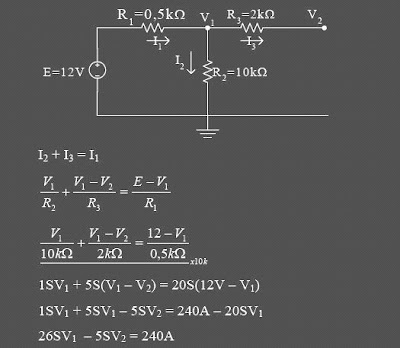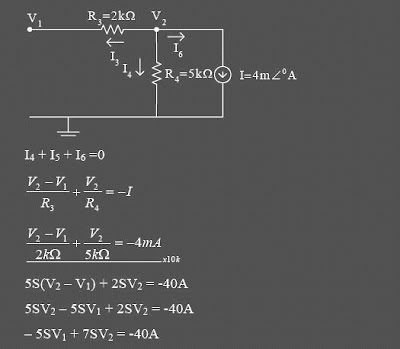## Wednesday, July 22, 2009

### Analisis Nodal/Simpul/Titik -Analisa Rangkain Elektronika

Analisis Nodal/Simpul/Titik Untuk mempermudah Analisa Rangkain Elektronika

Nodal / Simpul /titik Analysis By using this method, we will first obtain the size of the voltage on each of a series in which later can be used to explore the amount of electricity the other.

Stages to implement a Nodal / Simpul / Titik Analysis is this:
1. Determine the amount of twist in the series.
2. Choose a Simpul reference mark and the rest with a certain voltage, such as V1, V2, V3 and so on.
3. Determine the direction of flow on each knot. On a note that is not the directions, the directions are usually chosen exit / leave the simpul. Rename the current I1, i2, I3, and so on.
4. Apply Kirchoff current law at every twist in the simpul, except reference. Consider that all flows that do not know the directions, will leave every simpul.
5. Complete equality of linear simultan

Example
From the following series, specify the size of the voltage is in each simpul it!Determine the number of simpul:Simpul V1:Simpul V2:Expressed in the form of a matrix:Skema Rangkaian Elektronika